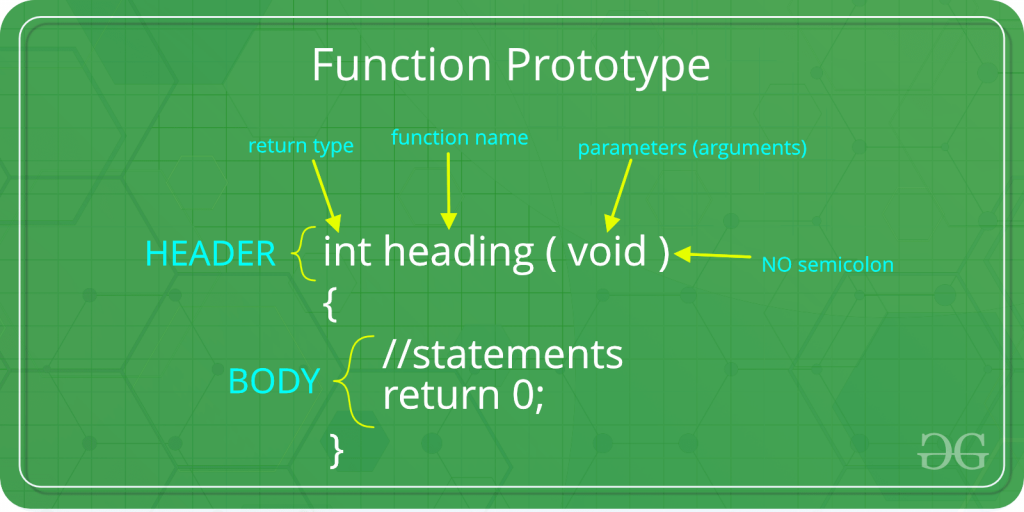# Argument Coercion in C/C++

• Difficulty Level : Basic
• Last Updated : 26 Dec, 2018

Argument coercion is a feature of function prototypes by which the compiler implicitly converts the datatype of the arguments passed during the function call to match the datatype in the definition of the function.Want to learn from the best curated videos and practice problems, check out the C++ Foundation Course for Basic to Advanced C++ and C++ STL Course for foundation plus STL.  To complete your preparation from learning a language to DS Algo and many more,  please refer Complete Interview Preparation Course.

It follows Argument promotion rules. Therefore a lower datatype maybe converted into a higher datatype but vice-versa is not true. This is because when a higher datatype is converted to a lower datatype it can result into loss or truncation of data.

The Promotion hierarchy for fundamental datatypes in C++ is:Example: Take for Example the code given below consists of an add function that expects double arguments. But even when integer arguments are passed it works correctly. In case long double arguments are passed the code will give an error.

 `#include ``using` `namespace` `std;`` ` `double` `add(``double` `a, ``double` `b)``{``    ``return` `a + b;``}`` ` `int` `main()``{``    ``// Passing double arguments, as expected``    ``cout << ``"Sum = "` `<< add(2.4, 8.5) << endl;`` ` `    ``// Passing int arguments, when double is expected``    ``// This will lead to Argument Coercion``    ``cout << ``"Sum = "` `<< add(16, 18) << endl;`` ` `    ``return` `0;``}`
My Personal Notes arrow_drop_up